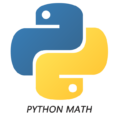# Test your knowledge of Python MathTotal Question : {{qCount}}
This QCM is designed to evaluate your knowledge of Python Math. It contains 10 questions and answers related to the subject. The questions cover topics such as addition, subtraction, multiplication, division, exponentiation, and absolute values. The answers are multiple choice and the correct answer is indicated by a hashtag. This QCM is a useful tool to quickly assess your understanding of math in Python.

## Test your knowledge of Python Math

{{(qIndex/questinnaire.questions.length)*100}}%